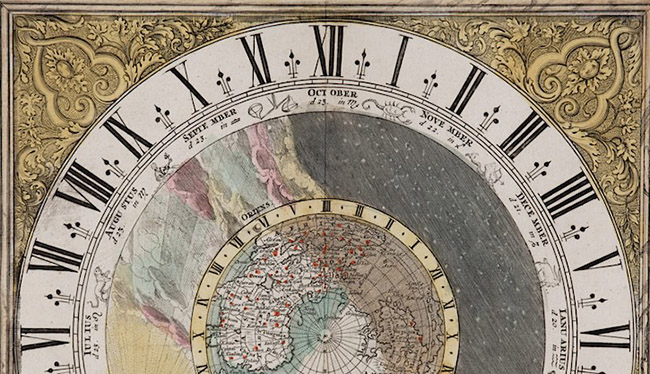# The Complete Guide to Roman Numerals### Tristan HughesHISTORYHIT.TV A new online only channel for history lovers

Despite its heyday occurring nearly 2,000 years ago, the legacy of ancient Rome still looms large all around us: in government, law, language, architecture, religion, engineering and art for instance.

One such area where this is especially true is Roman numerals. Today this ancient arithmetic system remains prevalent in various aspects of society: on clock-faces, in chemistry formulae, at the beginning of books, in the names of popes (Pope Benedict XVI) and monarchs (Elizabeth II).

Knowing Roman numerals thus remains useful; so here is your complete guide to Roman arithmetic.Waterloo Station’s famous clock face is one of many that predominantly uses Roman numerals. Credit: David Martin / Commons.

I = 1

V = 5

X = 10

L = 50

C = 100

D = 500

M = 1,000

## Higher + lower

The Roman equivalent to any number that did not equal one of the above values was made by combining two of more of these symbols.

In most cases the symbols would be added together, starting with the symbol that had the highest value on the left and ending with the lowest on the right.

8 in Roman numerals, for instance, is VIII (5 + 1 + 1 + 1).

782 is DCCLXXXII (500 + 100 + 100 + 50 + 10 + 10 + 10 + 1 + 1).

1,886 is MDCCCLXXXVI (1,000 + 500 + 100 + 100 + 100 + 50 + 10 + 10 + 10 + 5 + 1).

## The exceptions

There are a couple of occasions when a lower value Roman numeral will appear before a higher and in this case you subtract the lower value from the higher directly after it.

4 for instance is IV (5 – 1).

349 is CCCXLIX (100 + 100 + 100 + 50 – 10 10 – 1).

924 is CMXXIV (1,000 – 100 + 10 + 10 + 5 – 1).

1,980 is MCMLXXX (1,000 + 1,000 – 100 + 50 + 10 + 10 + 10).

A lower value will only appear in front of a higher value Roman numeral when either the number 4 or the number 9 is included.

## Numeral endings and overlines

Roman numerals usually end with a symbol between I and X.

349, for instance, would not be CCCIL (100 + 100 + 100 + 50 – 1) but CCCXLIX (100 + 100 + 100 + 50 – 10 + 9).

To express numbers above 3,999 (MMMCMXCIX) in a more convenient way, by the Middle Ages Roman numerals could be multiplied by 1,000 by adding an overline to the numeral.

It is debated, however, whether this system was employed by the Romans or whether it was only added later, during the Middle Ages.

## Key Roman numerals from 1 – 1,000

I = 1

II = 2 (1 + 1)

III = 3 (1 + 1 + 1)

IV = 4 (5 – 1)

V = 5

VI = 6 (5 + 1)

VII = 7 (5 + 1 + 1)

VIII = 8 (5 + 1 + 1 + 1)

IX = 9 (10 – 1)

X = 10

XX = 20 (10 + 10)

XXX = 30 (10 + 10 + 10)

XL = 40 (50 – 10)

L = 50

LX = 60 (50 + 10)

LXX = 70 (50 + 10 + 10)

LXXX = 80 (50 + 10 + 10 + 10)

XC = 90 (100 – 10)

C = 100

CC = 200 (100 + 100)

CCC = 300 (100 + 100 + 100)

CD = 400 (500 – 100)

D = 500

DC = 600 (500 + 100)

DCC = 700 (500 + 100 + 100)

DCCC = 800 (500 + 100 + 100 + 100)

CM = 900 (1,000 – 100)

M = 1,000

For all big pub quizzers out there we are now in the year MMXVIII, soon to be MMXIX.